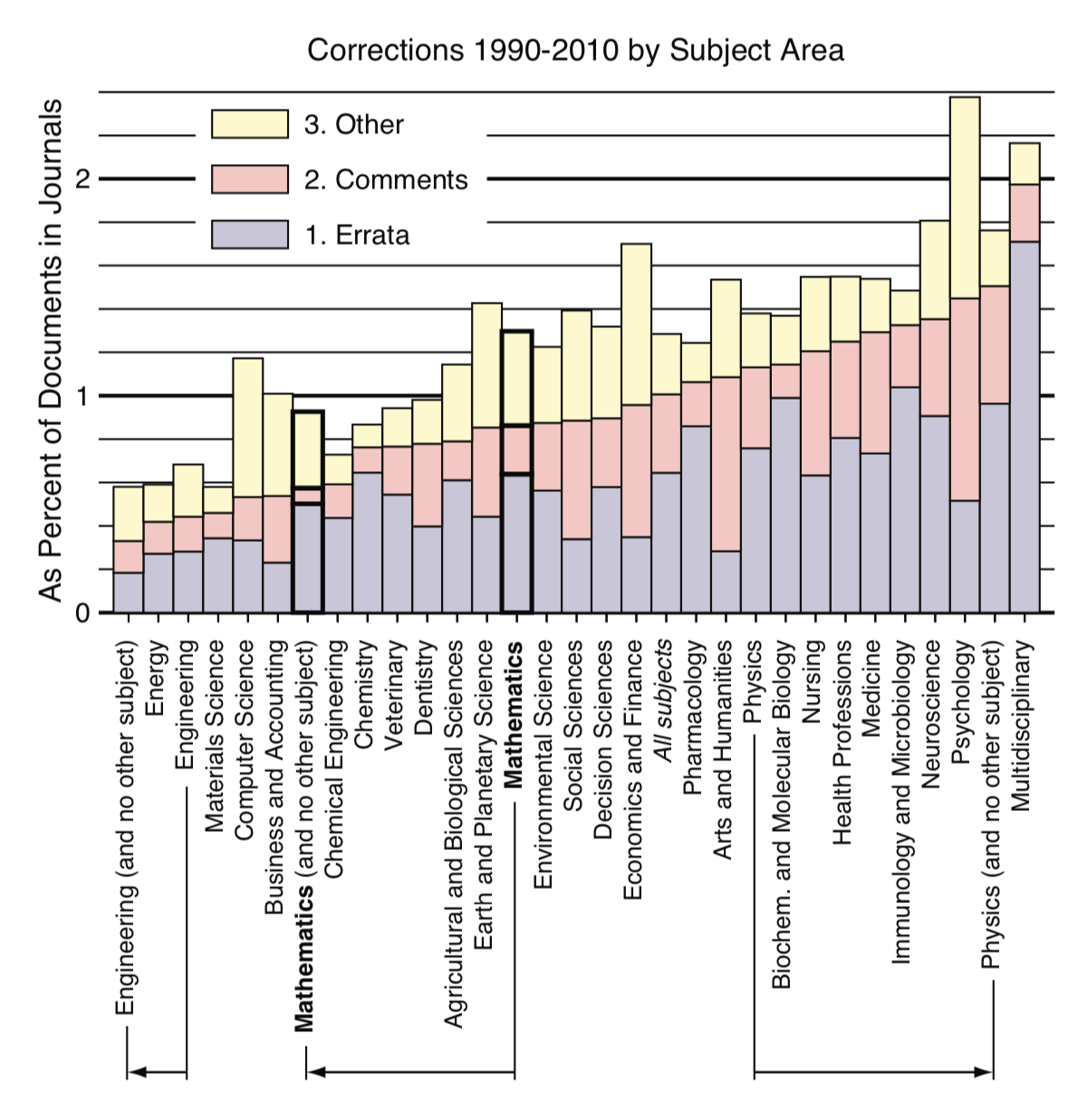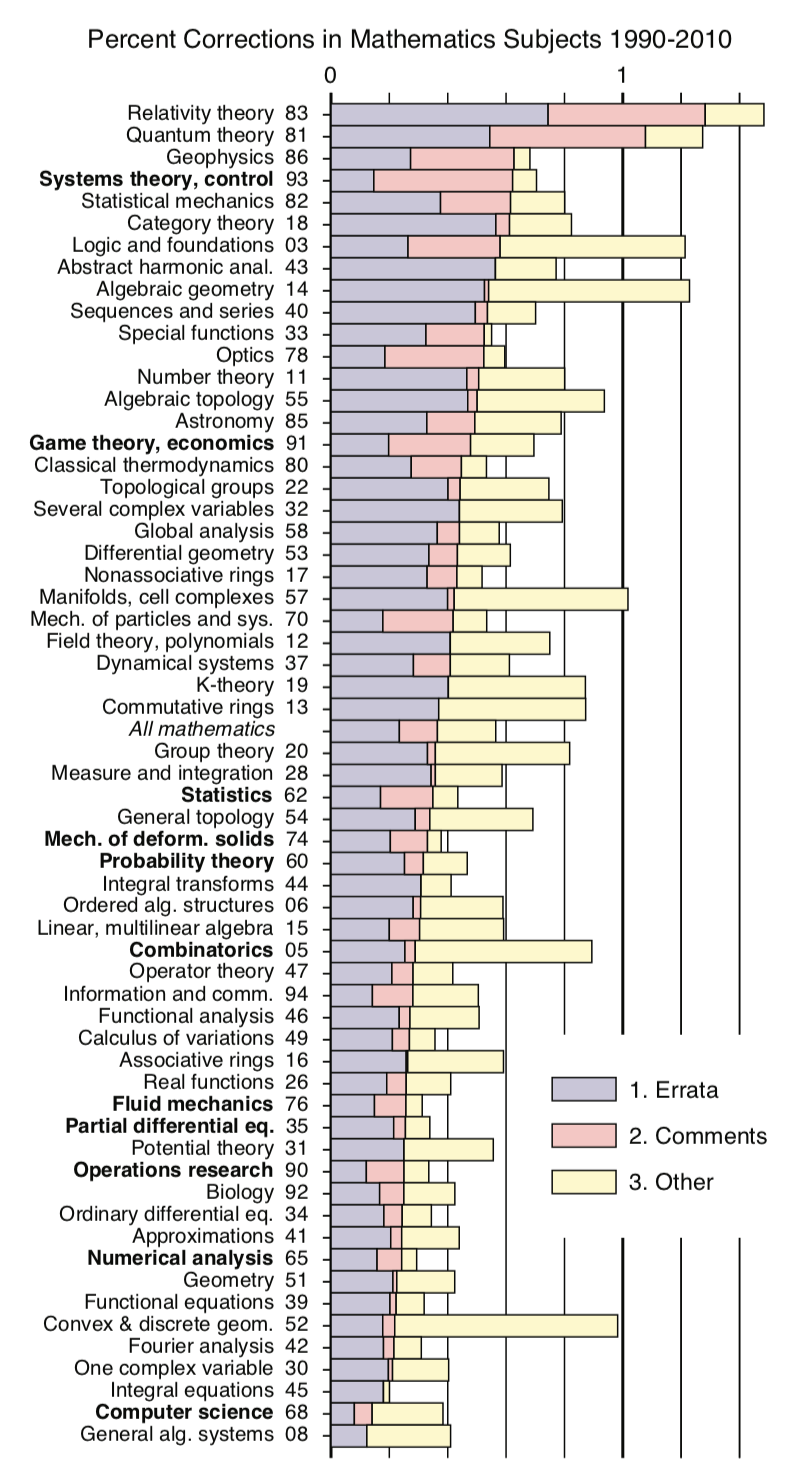# What percentage of published mathematics papers are correct?

As described in Larcombe and Ridd, estimates for the percentage of published papers found to be reliable or reproducible in sciences such as biomedical science, have been as low as 50%, 25% and even 11%.

My question is: In mathematics, what percentage of published papers are correct? (i.e. all new theorems presented in the paper are correct, and can thus be relied on). Have any studies been done? Perhaps estimates could be made, based on the number of published corrections?

Note that while the problem of finding the exact answer to this question, for some given set of journals, might be beyond our present capability, the question itself, is objective - not a matter of opinion.

• This is an interesting question, but there is no way to answer. 1. A published correction does not mean that the paper is incorrect. 2. Not all authors care to publish corrections. And how do we know even that one, particular paper is correct? If many people read it carefully, this only suggests that it is probably correct. But most mathematical papers are not read by anyone. Feb 1 '18 at 6:14
• The answer must surely depend on what "correct" means. If a key line in a lemma says "5+3+7 = 7+7 = 15", and the author subsequently uses only the consquence that 5+3+7 = 15, is the paper incorrect? Feb 1 '18 at 6:29
• If you mean every claim and every step of the argument is correct, approximately 0% - the same as asking what percentage of computer programs in use have no bugs. If you mean that the main theorem is true, I think a clear majority. If you mean that the main theorem is true after minor modification, probably a vast majority. Feb 1 '18 at 7:05
• Of course, we have no idea which percentage of papers in the sciences are correct, only the percentage that are reproducible. If someone succesfully reproduces a paper using the same method, they could be making the same mistake. A good analogue of replication in mathematics is when someone needs to prove a generalization of a result, so they read the paper and then adapt the argument to the new setting - i.e. they reproduce the argument based on the description in the paper. Attempts to do this often turn up some minor flaw in the original paper and occasionally turn up a serious flaw. Feb 1 '18 at 7:08
• So I would say most papers in math are replicable in the sense that another mathematician, reading the papers carefully, and applying their own standard of rigor, would be able to prove (or convince themselves that they've proved) the same result. Feb 1 '18 at 7:08

This graph from Errors and Corrections in Mathematics Literature indicates about 1.4% of published mathematics papers were followed by a correction.Corrections as percent of journal documents for subject areas of Scopus. Three areas are shown both inclusive and exclusive of interdisciplinary work. Comments are undercounted for journals that choose to identify them as “letters”.

There is also a breakdown per mathematical topic:Error correction rates as percent of articles in the primary Mathematics Subject Classifications from Zentralblatt. The eleven subjects in bold contribute 51 percent of publications.

• In this (well worth reading) paper, an important point is made: there is a significant rift between applied and pure mathematics, including traditions, attitudes, methodology and so on. Because of this, it is not reasonable to lump all of mathematics together, as all the statistics relevant to pure math will be buried in a much bigger applied pile. Feb 2 '18 at 11:48
• By the way, I completely agree with the main point: even if any expert knows that a certain paper is wrong, it is very unlikely for them to do something about it. This makes life pretty hard for a beginner; good thing now we have MO. Feb 2 '18 at 11:55
• I find it amusing that Logic and foundations is near the top of the list. Feb 14 '18 at 9:57
• This percentage is of little relevance, for plenty of reasons. Why the same weight for each paper? Also a paper can have 5 independent main theorems, one of which is false. And "followed by a correction", how is it counted? it can be mentioned as false in one or several papers by other authors, and then these statistics will most likely not take this into account. Finally, even for a given theorem, "false" has plenty of meanings, with different consequences, as was already said in the comments.
– YCor
Feb 14 '18 at 10:15
• thanks for the feedback, @PeterHeinig , I changed "error rates" into "error correction rates" as you advised. Feb 15 '18 at 14:41